﻿ Graph Analytics Tutorial with Spark GraphX | Aegis
Skype

Graph Analytics Tutorial with Spark GraphX

Quick InquiryYou Are @ >> Home >> Articles >> Graph Analytics Tutorial with Spark GraphX

Relationships between data can be seen everywhere in the real world, from social networks to traffic routes, from DNA structure to commercial system, in machine learning algorithms, to predict customer purchase trends and so on. Graph analytics can make sense of these connections to derive some incredible outputs and to provide more insight that cannot see with naked eyes. It widely used for recommendation engines, fraud detection, route optimization, social network analysis, page ranking, and many more. In this blog, we are going to discuss in detail one of the most popular graph analytics computing framework- Spark GraphX.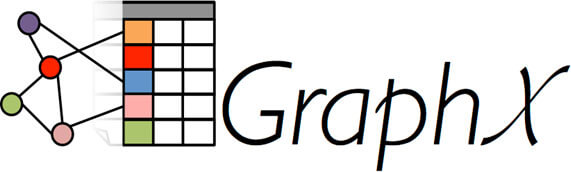Different graph computing engines

There are many graph analytics frameworks in the market - Apache Girafe, Pregel, GraphLab, Spark GraphX.These graph engines provide an optimized abstraction over various graph algorithms, which can be run in a distributed environment thus processing large graphs in parallel and fault-tolerant manner.

Spark Graphx

Spark GraphX is a distributed graph computing engine that extends Spark RDD. How it is different from other graph-processing framework is that it can perform both graph analytics and ETL and can do graph analysis on data that is not in graph form. Spark Graphx provides an implementation of various graph algorithms such as PageRank, Connected Components, and Triangle Counting.

GraphX supports property multigraph, which is a directed graph with multiple parallel edges to represent more than one relationship between the same source node & destination node. For example, a person can stay & work from the same address, which means the same address can use as a home address as well as an office address for that, the person thus representing multiple relationships.

• Property graphs are immutable just like RDD, which means once we create the graph it cannot be modified but, we can transform it to create new graphs.
• Property graphs distributed on multiple machines (executors) for parallel processing.
• Property graphs are fault-tolerant, which means it can recreate in case of any failures.
• In Spark GraphX, nodes and relationships are represented as dataframes or RDDS. Node dataframe must have a unique id along with other properties and the relationship dataframe must have a source and destination id along with other attributes.
• For example, we have a graph where nodes represent the cities name of the United States along with the average temperature, and edges represent much flight between these cities.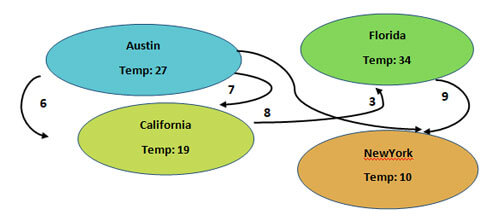Dataframe to represent Nodes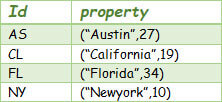Dataframe to represent Relationship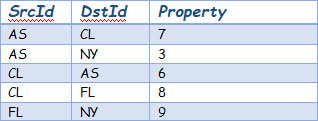To get started with the graphX project, we need to import spark packages into our project.

importorg.apache.spark._ importorg.apache.spark.graphx._ importorg.apache.spark.rdd.RDD

Secondly, we need to create RDD’s/Datframes from data and then create a graph from those RDD. Let us create a graph from the above example of cities in the united stated and their connected flights.

val citiesArray = Array( (1L,("Austin", 27)), (2L,("California", 19)), (3L,("Florida", 34)), (4L,("NewYork", 10)) ) val flightsArray = Array( Edge(1L,2L,7), Edge(1L,4L,3), Edge(2L,1L,6), Edge(2L,3L,8), Edge(3L,4L,9) ) val cityNodeRDD:RDD[(VertexId,(String,Int))] = sc.parallelize(citiesArray) val flighEdgeRDD: RDD[Edge[Int]] = sc.parallelize(flightsArray) val graph: Graph[(String, Int), Int] = Graph(cityNodeRDD, flighEdgeRDD)

Once we have data represented in the form of a graph, we can do filtering, joining, mapping and can apply numerous graph operators available in spark graphx library.

Let’s filter out cities which has an average temperature less than 24

graph.vertices.filter {case (id, (name, temp)) => temp < 24} graph.edges.filter {case Edge(src,dst,flights) =>flights>5}

Let us filter out all flight routes where the frequency of flights is more than five

Apart from the nodes and edges view, graphx also has a triplet view, which joins nodes and edges properties resulting in EdgeTriplet RDD (SourceId, SrcAttributes, DestinationId, DestinatioAttributes, and EdgeAttributes).

Below is the triplet for above graph

• ((1,(Austin,27)),(2,(California,19)),7)
• ((1,(Austin,27)),(4,(NewYork,10)),3)
• ((2,(California,19)),(1,(Austin,27)),6)
• ((2,(California,19)),(3,(Florida,34)),8)
• ((3,(Florida,34)),(4,(NewYork,10)),9)There are several graphx operators are used to do computations with graphs. We will look at a few of them in this blog and will answer some interesting analytics about flight data.

//Total Number of flight routes graph.numEdges Long = 5 //Total Number of cities in United States graph.numVertices Long = 4 //Number of incoming flight into a city graph.inDegrees (4,2) // (cityid,number of flights landing into city airport) (1,1) (3,1) (2,1) //Total number of incoming as well as outgoing flight from a city graph.degrees (4,2) //(cityid,total number of incoming & outgoing flight from a city) (1,3) (3,2) (2,3)

Subgraphs

Using graph operators, we can also create subgraphs out of a graph using some predicates on vertexes or edges. Let us say we have a graph that represents the people working in their organization and their relationship based on their designation. Using the subgraph operator, we will create a subgraph that will only have a manager as the relationship.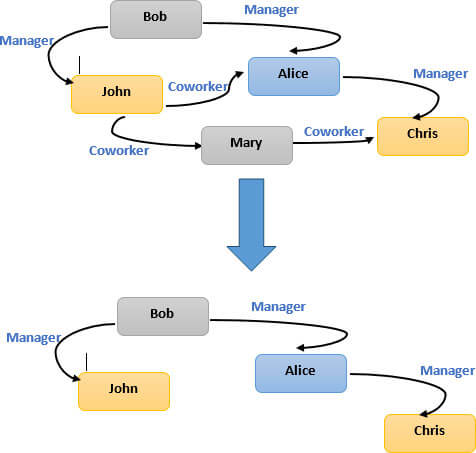valmanagerGraph=graph.subgraph(epred= (edge)=>edge.attr =="Manager")

Caching/Uncaching

To avoid recomputation in case of failures, graphs can be cached using Graph.cache() method so that the graph can be recreated and can exist in memory for fast computation. In the case of iterative computations, the intermediate results from the previous iteration can fill up the cache fast so to obtain the best performance we may need to uncache the graph. In the case of iterative computations, you should use the Pregel API that unpersists intermediate results correctly.

GraphX Algorithms

PageRank

GraphX provides an implementation of various graph algorithms, such as PageRank. PageRank measures the importance of each vertex in a graph.

For example, in-flight example, if a city has a higher number of incoming & outgoing flights then, that city might have higher importance than other cities. Graphx provides dynamic, as well as the static implementation of PageRank. In a static implementation, the algorithm will run a fixed number of times, whereas in dynamic implementation; Pagerank will run until the ranks converge.

val ranks = graph.pageRank(0.0001).vertices

Connected Components

A Connected Component is a connected subgraph where two vertices connected by an edge, and there are no additional vertices in the main graph. This algorithm is also used to label each connected component of the graph with the ID of its lowest-numbered vertex.

valconnectedComponents= graph.connectedComponents().vertices

Triangle Counting

Triangle counting is used to detect community in a graph by determining the number of triangles passing through each vertex. This algorithm heavily used, in social network analysis spam detection and link recommendations.

val triCounts = graph.triangleCount().vertices

Conclusion

In this article, Apache Spark Integration expert shows you how Spark graphx provides a simple and optimized interface for graph processing and is faster as compared to other graph processing engines because of its in-memory computation capability. Support to perform exploratory as well as graph analysis on the same data makes this graph computing framework even more interesting.

For further information, mail us at info@aegissoftwares.com